Chapter OverviewMaths

Exam board

Pearson Edexcel

Number

Algebra

Graphs

Ratio proportion and rates of change

Shapes and area

Angles and geometry

Trigonometry

Probability

Statistics

Maths

# Scatter graphs0%

Summary

# Scatter graphs

## ​​In a nutshell

A scatter graph is a graph displaying plotted data points.  The graph tells you how closely variables are related to each other as well as the kind of relation they have.

## Interpreting scatter graphs

Scatter graphs tell you about correlation between variables. They do not prove that one variable has an effect on another - correlation could happen because of a third factor or just by coincidence.

### Definitions

 Correlation How closely two variables are related Outliers Points on the graph that do not fit the trend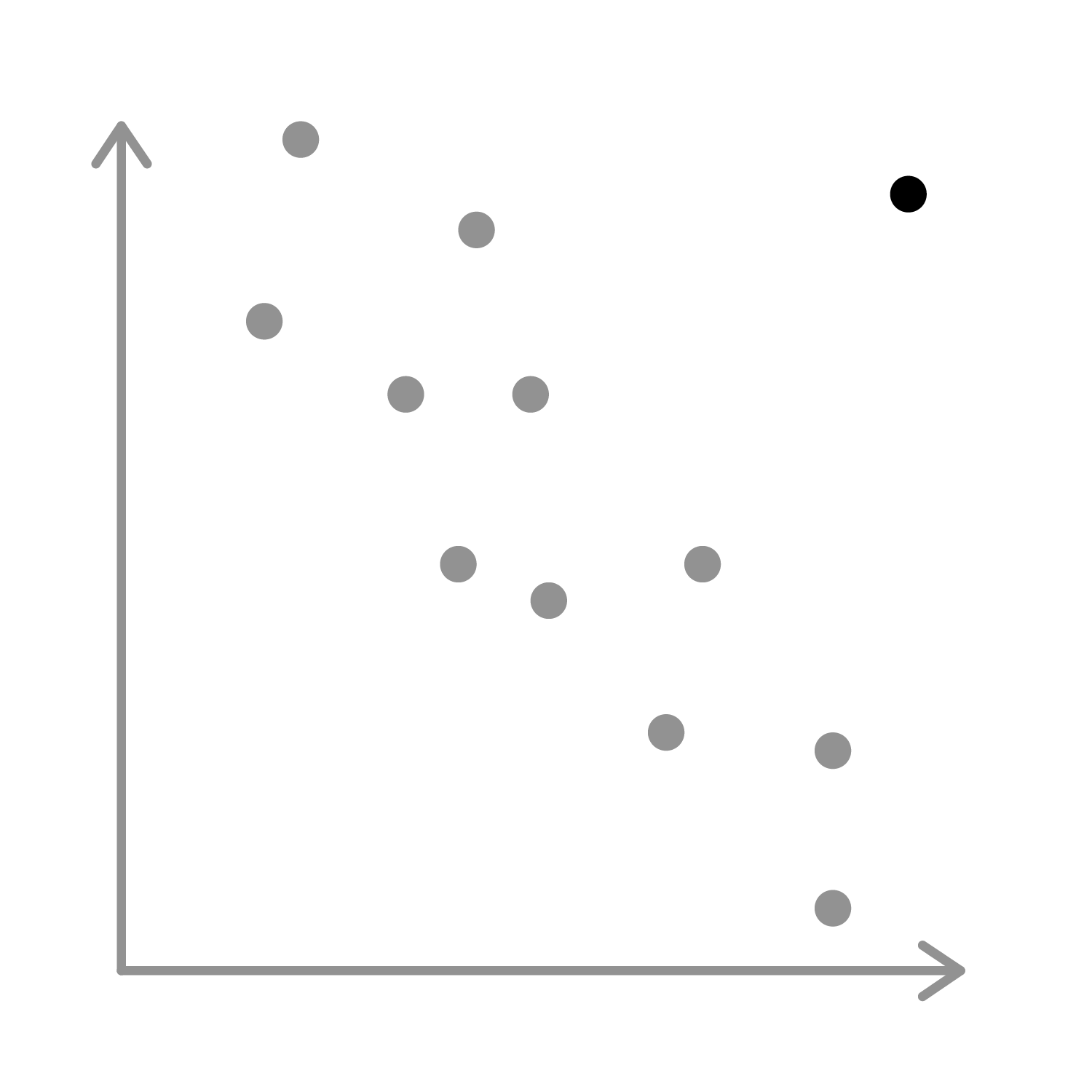Line of best fit A straight line drawn through the middle of points, as close to each one as possible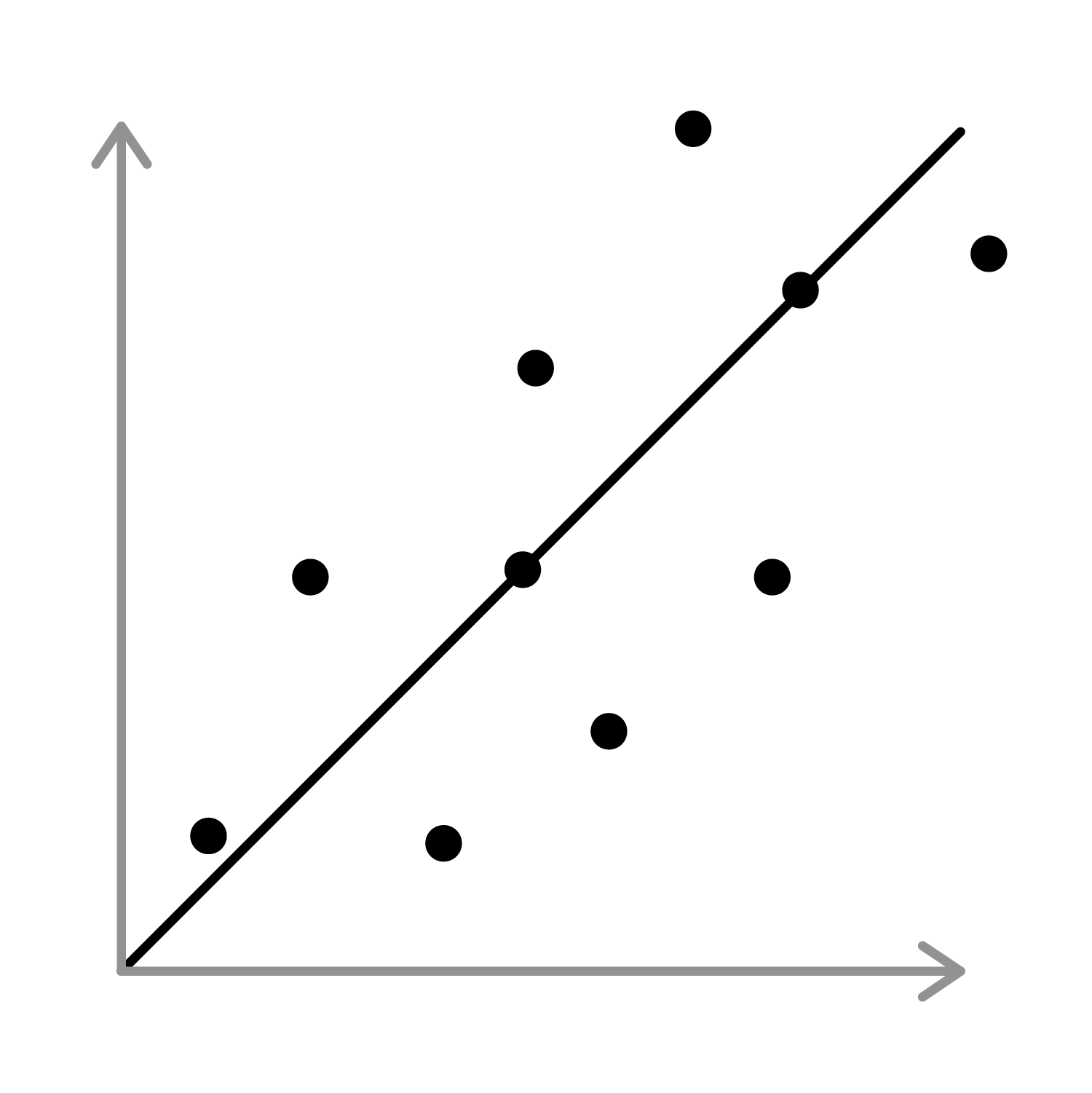Strong correlation Points make a fairly straight line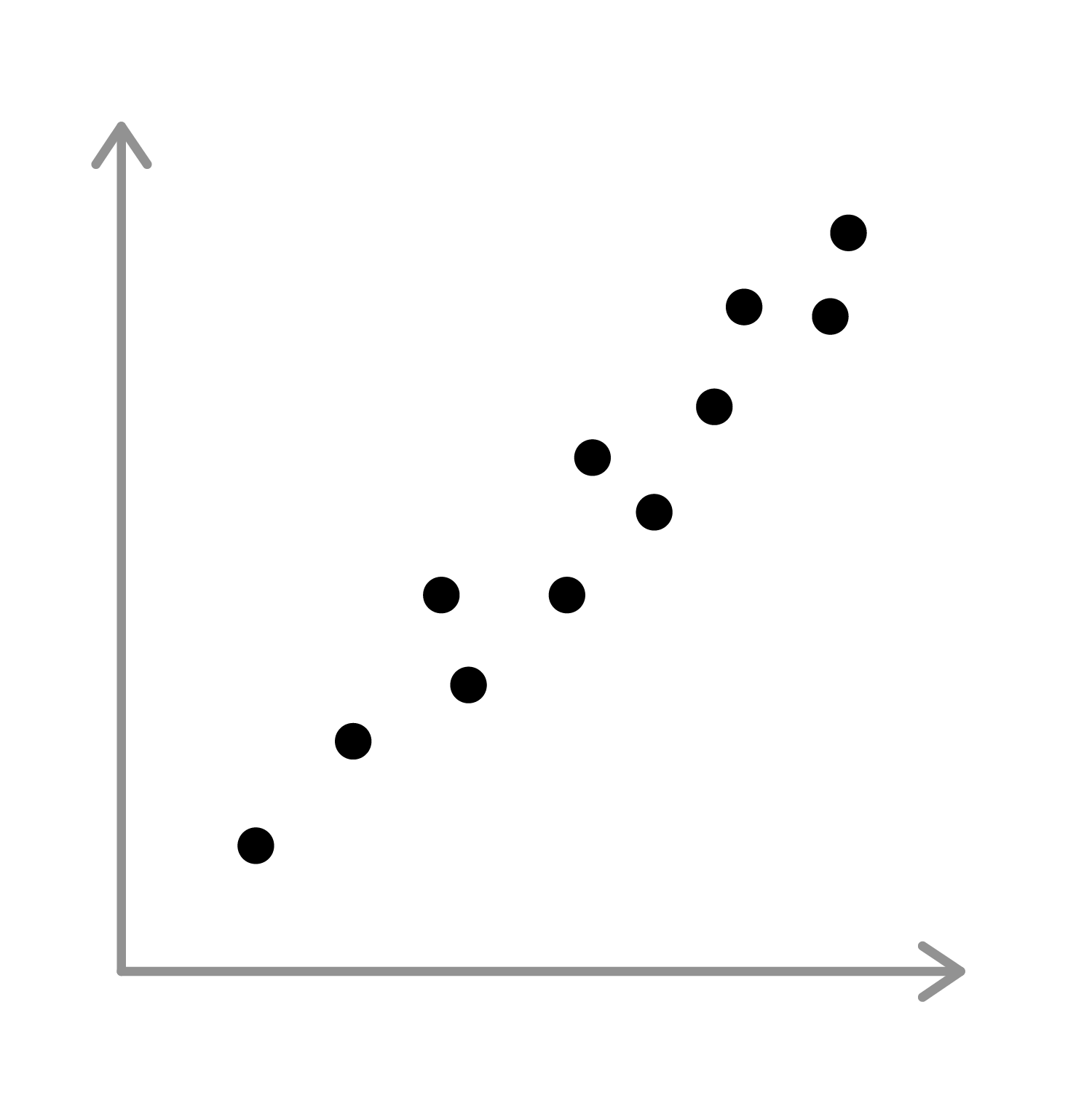Weak correlation Points stray more from a straight line, but there is still positive or negative correlation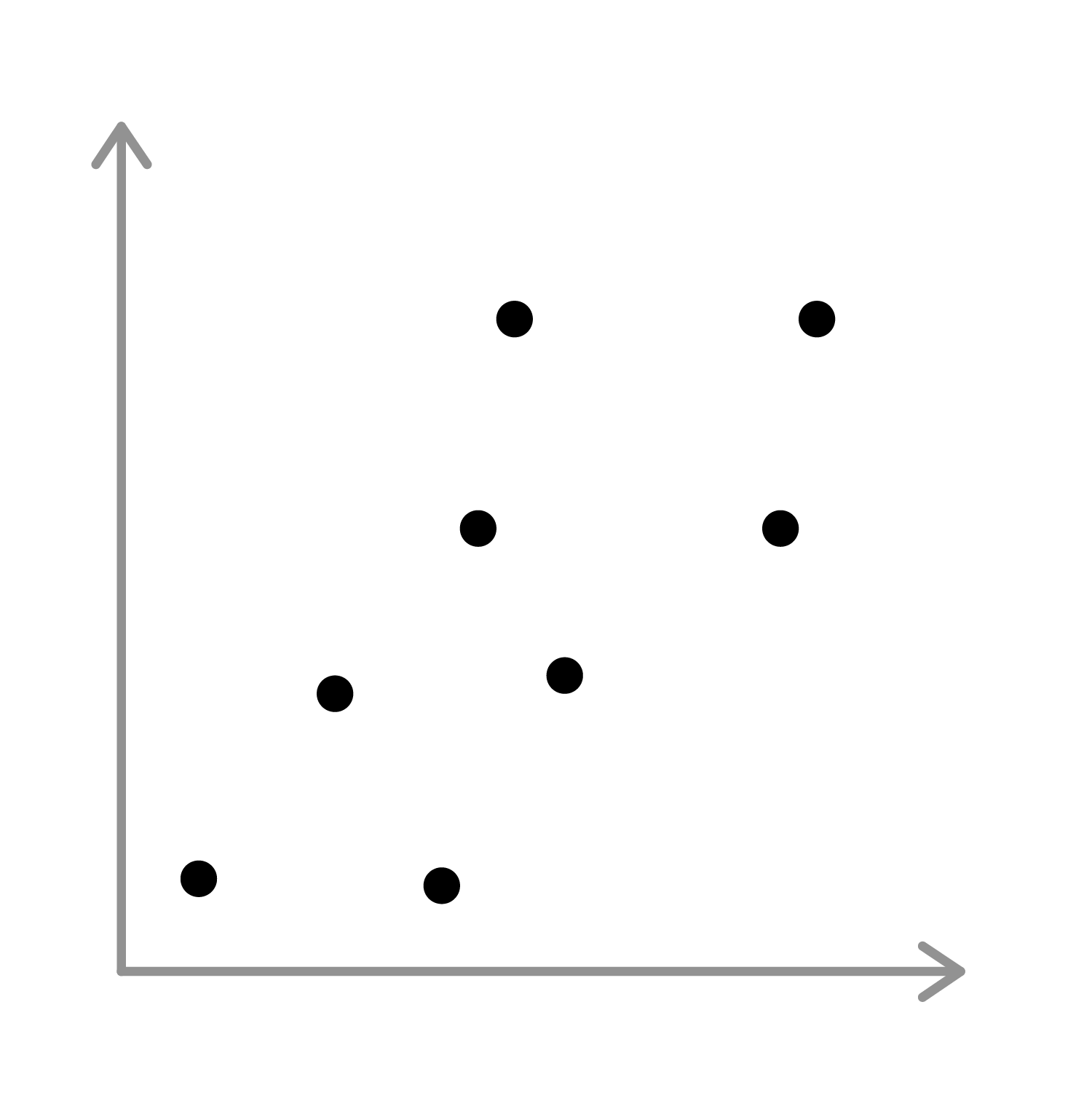Negative correlation Sloping downhill from left to right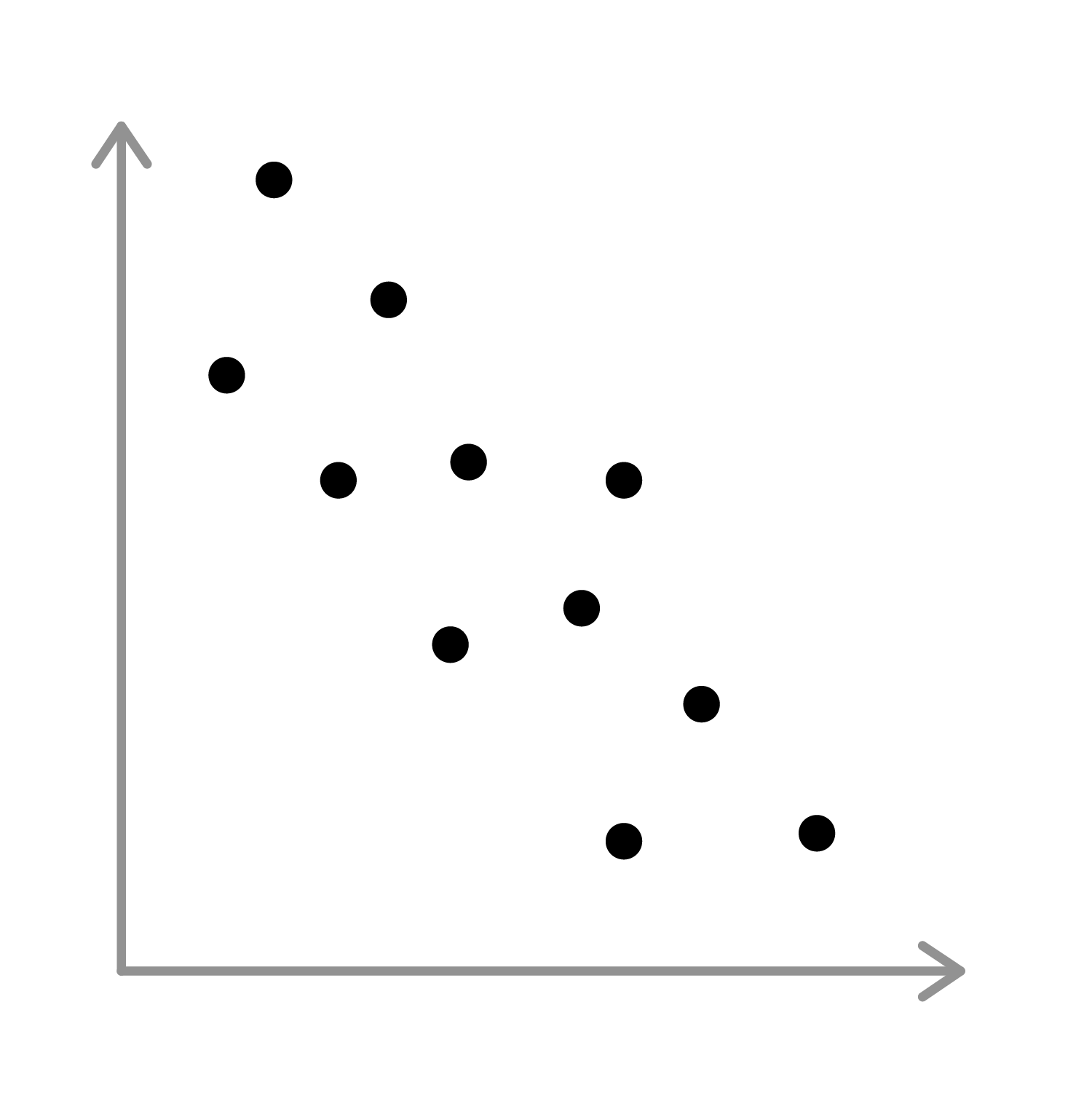Positive correlation Sloping uphill from left to rightNo correlation Points have no pattern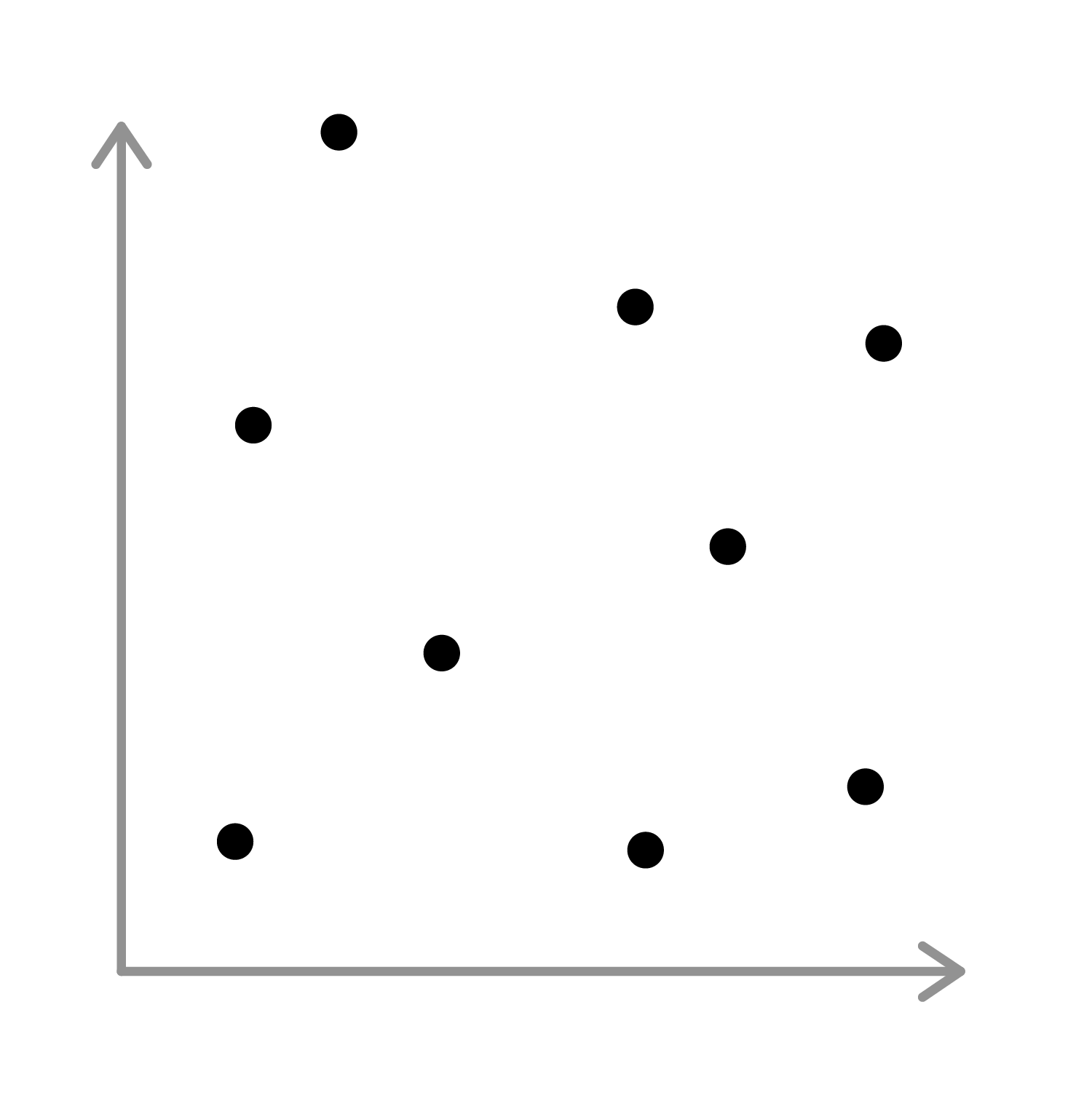### Making predictions using scatter graphs

By drawing a line of best fit, you can make predictions using scatter graphs. It is important when drawing a line of best fit to ignore any outliers, as these could be potentially caused by an error that occurred during the experiment or when recording the data.

Tip: When drawing a line of best fit, make sure there are the same amount of points above the line as there are below.

##### Example 1

This scatter graph shows the correlation between the number of books read per year and age.

Draw a line of best fit and predict how many books per year a thirty-five year old would read.

Reading up and along, you can predict that a thirty-five year old would read $\underline {16 }$ books a year.

Note: In the example above, the line of best fit has been 'extrapolated' to make a prediction about the future.

## Accuracy of predictions

 Extrapolation A type of estimation which goes outside the original range of observed or recorded values, based on the trends in the previous results.

Whilst this does provide a useful prediction, it is not always reliable as you have no way of knowing whether the current trend in data will remain the same.

## Want to find out more? Check out these other lessons!

Scatter graphs

FAQs

• Question: What is weak correlation?

Answer: Weak correlation is where the points plotted stray more from a straight line, but there is still positive or negative correlation.

• Question: What is strong correlation?

Answer: Strong correlation is where the points make a fairly straight line.

• Question: What is a line of best fit?

Answer: A line of best fit is a straight line drawn through the middle of points, as close to each one as possible.

• Question: What is an outlier point?

Answer: Outlier points are points on the graph that do not fit the trend.

• Question: What is a scatter graph?

Answer: A scatter graph is a graph showing plotted points that tells you how closely things are related to each other as well as the kind of relation they have.

Theory

Exercises